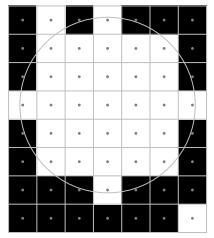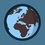# Moon

Dasha got a new camera. She taked some photo about the moon, but the resolution was not too hight. In the photo only two colors are visible, white and black.

Dasha taked a lot of picture and she want to know where is the position of the moon in each photo. The moon has an r radius$(r\geq0)$. The Moon is a set of squares, where each square is colored white from the center of the moon in r range. So if a block's center is in the Moon, then the block should be colored white.Unfurtunetly some stars are visible on the picture.

Write a program which print the largest possible radius of the Moon, and print out the position of the Moon.

#### Input

Two integer, w and h- the width and the height of the picture$(1\leq w,h\leq 50)$. In the next h lines contain w characters. . means black and * means white squares.

#### Output

First line should contain the largest possible radius. The next line should contain the coordinates of the Moon's center(column, row, numbered from 1).

If there are more possible outputs, then print only the first. Left to Right, Up to Down.

$\color{#888888}\Large\bigodot \hspace{-666200sp}\rule[3px]{1.3px}{5.5px}\hspace{-289000sp}\rule[11.5px]{7.5px}{1.7px}$ $\hspace{1pt}$ Time limit 1 second

$\color{#888888}\rule[4px]{14px}{2.2px}\hspace{-14px}\rule{14px}{2.2px}\hspace{-14px}\rule[-4px]{14px}{2.2px}$ Memory limit 64 MiB

$\begin{array}{ll} \textsf{\large Input example \#1}\hspace{200px}&\textsf{\large Output example \#1}\\ \begin{matrix} \texttt{7}&\texttt{8}\\ \texttt{.}&\texttt{*}&\texttt{.}&\texttt{*}&\texttt{.}&\texttt{.}&\texttt{.}\\ \texttt{.}&\texttt{*}&\texttt{*}&\texttt{*}&\texttt{*}&\texttt{*}&\texttt{.}\\ \texttt{.}&\texttt{*}&\texttt{*}&\texttt{*}&\texttt{*}&\texttt{*}&\texttt{.}\\ \texttt{*}&\texttt{*}&\texttt{*}&\texttt{*}&\texttt{*}&\texttt{*}&\texttt{*}\\ \texttt{.}&\texttt{*}&\texttt{*}&\texttt{*}&\texttt{*}&\texttt{*}&\texttt{.}\\ \texttt{.}&\texttt{*}&\texttt{*}&\texttt{*}&\texttt{*}&\texttt{*}&\texttt{.}\\ \texttt{.}&\texttt{.}&\texttt{.}&\texttt{*}&\texttt{.}&\texttt{.}&\texttt{.}\\ \texttt{.}&\texttt{.}&\texttt{.}&\texttt{.}&\texttt{.}&\texttt{.}&\texttt{*} \end{matrix}& \begin{matrix} \texttt{3}\\\texttt{4}&\texttt{4}\\ \\ \\ \\ \\ \\ \\ \\ \end{matrix} \end{array}$

From there

Bonus problem: Write a program, which print the Moon with a given radius.

# Factorisation

One number, n.

#### Output

Some lines. The kth line contains the amount of numbers with k prime factors 2 to n. The lines can't contain 0.

No limits

$\begin{array}{ll} \textsf{\large Input example \#1}\hspace{200px}&\textsf{\large Output example \#1}\\ \begin{array}{l} \texttt{10}\\ \\ \end{array}& \begin{array}{l} \texttt{4}\\\texttt{4}\\\texttt{1} \end{array}\\ \textsf{\large Input example \#2}\hspace{200px}&\textsf{\large Output example \#2}\\ \begin{array}{l} \texttt{10000}\\ \\ \\ \\ \\ \\ \\ \\ \\ \\ \\ \\ \end{array}& \begin{array}{l} \texttt{1229}\\\texttt{2625}\\\texttt{2569}\\\texttt{1712}\\\texttt{963}\\\texttt{485}\\\texttt{231}\\\texttt{105}\\\texttt{47}\\\texttt{22}\\\texttt{7}\\\texttt{3}\\\texttt{1} \end{array} \end{array}$

# SolutionsNote by Páll Márton (no activity)
2 months, 4 weeks ago

This discussion board is a place to discuss our Daily Challenges and the math and science related to those challenges. Explanations are more than just a solution — they should explain the steps and thinking strategies that you used to obtain the solution. Comments should further the discussion of math and science.

When posting on Brilliant:

• Use the emojis to react to an explanation, whether you're congratulating a job well done , or just really confused .
• Ask specific questions about the challenge or the steps in somebody's explanation. Well-posed questions can add a lot to the discussion, but posting "I don't understand!" doesn't help anyone.
• Try to contribute something new to the discussion, whether it is an extension, generalization or other idea related to the challenge.

MarkdownAppears as
*italics* or _italics_ italics
**bold** or __bold__ bold
- bulleted- list
• bulleted
• list
1. numbered2. list
1. numbered
2. list
Note: you must add a full line of space before and after lists for them to show up correctly
paragraph 1paragraph 2

paragraph 1

paragraph 2

[example link](https://brilliant.org)example link
> This is a quote
This is a quote
    # I indented these lines
# 4 spaces, and now they show
# up as a code block.

print "hello world"
# I indented these lines
# 4 spaces, and now they show
# up as a code block.

print "hello world"
MathAppears as
Remember to wrap math in $$ ... $$ or $ ... $ to ensure proper formatting.
2 \times 3 $2 \times 3$
2^{34} $2^{34}$
a_{i-1} $a_{i-1}$
\frac{2}{3} $\frac{2}{3}$
\sqrt{2} $\sqrt{2}$
\sum_{i=1}^3 $\sum_{i=1}^3$
\sin \theta $\sin \theta$
\boxed{123} $\boxed{123}$

Sort by:

My lame brute logic: try BFS from every *. Since a moon’s center is at an integer grid, we can find the grid through brute force.

Or apply Floodfill to find the regions. Then find the up and down, left and right limits to the region. then test a $3\times 3$ matrix center of grid $(U+D)\div 2,(L+R)\div 2$.

- 2 months, 4 weeks ago

Can you solve the second problem?

- 2 months, 3 weeks ago

Do the factors need to be different?

- 2 months, 3 weeks ago

I added now, you should see only the prime factors

- 2 months, 3 weeks ago

  1 2 3 4 5 6 7 8 9 10 11 12 13 14 15 16 17 18 19 20 21 22 23 24 25 26 27 #include #include //I’ll define the testing function here. //You know what? I’m typing the whole code! int n,match; int test(int x,int y,int tx,int ty,int a)//x-origin,tx-tested { int nx,ny;//the three rotated dots nx=tx;//org ny=ty; nx=ty-y+x;//clock90 ny=tx-x+y; nx=x-tx;//180 ny=y-ty; nx=y-ty+x;//clock270 ny=x-tx+y; if(nx==nx==nx==nx&&ny==ny==ny==ny)//match { match[n]=1; //test next grid } } int main { int row,col; int x; } 

- 2 months, 4 weeks ago

  1 2 3 4 5 6 7 8 9 10 11 from sympy import factorint from collections import defaultdict N = int(input()) count = defaultdict(int) for i in range(2, N+1): n = len(factorint(i, multiple=True)) count[n] += 1 for n in sorted(count.keys()): print(count[n]) 

- 1 month, 3 weeks ago

Thank you! I will try it.

- 1 month, 3 weeks ago

But wait, how do you define a moon’s radius? also if the stars are arranged around the moon, expanding it? :)

- 2 months, 4 weeks ago

The biggest perfect circle will be the Moon. So if one block is missing from a perfect circle, then it can't be the Moon. BTW you should write the whole code :)

- 2 months, 4 weeks ago

I am working on it, maybe finished by tomorrow :)

- 2 months, 4 weeks ago

BTW, have you found a solution?

- 2 months, 4 weeks ago

See the note again :)

- 2 months, 4 weeks ago

Nice :)

- 2 months, 4 weeks ago

Try these!

- 2 months, 4 weeks ago

Nice!

- 2 months, 4 weeks ago

Thank you!

- 2 months, 4 weeks ago